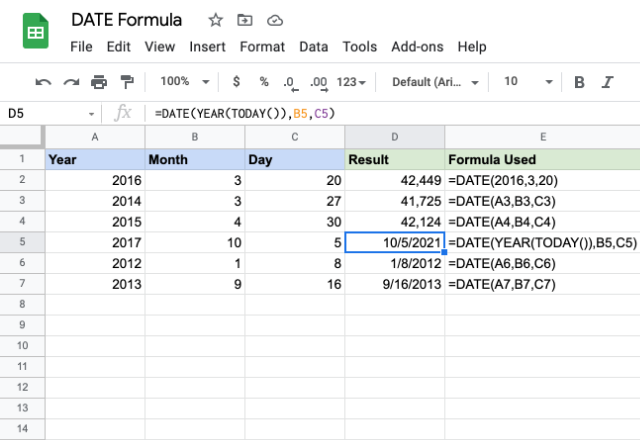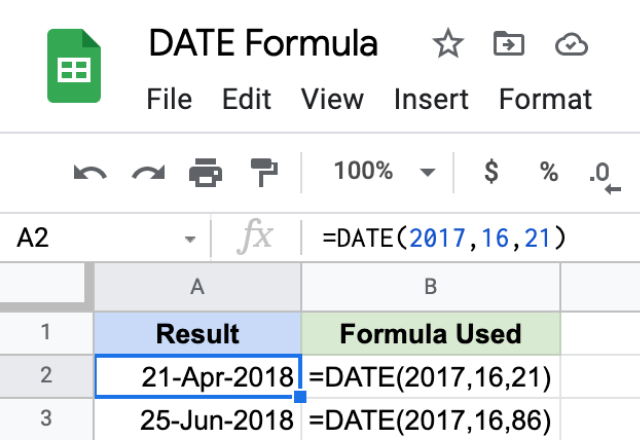# How to use the DATE formula in Google SheetsDates can behave badly in spreadsheets. Some date formats are not recognized and although you can use times and dates in formulas, you must ensure that the date is enclosed in quotes. Google Sheets has many time and date-related functions and the most basic of these is DATE.

Quite simply, you enter numbers representing a year, a month and a day, e.g. 1985, 12, 25 and the formula returns these values in a date format: 12/25/1985. 25th December 1985.

This seems so basic you might be wondering why you’d ever need this function at all. It’s more helpful than you might think:

#### Regional date formatting

Writing formats for dates varies from country to country. DATE standardizes this: you have to write the number of the year followed by the number of the month, followed by the number of the day. Note that you can still choose dates in your spreadsheet to be displayed however you like. Go to Format > Number > More formats > More date and time formats.

#### Include dates in formulas

Typing DATE into your formula is a quick and reliable way to tell Google Sheets that you are entering a date. An alternative is to use quotes but you must remember to include the quotes “_” correctly and enter the numbers in the correct order.

#### Quickly retrieve values from dates in large datasets

If you want to analyze a dataset that includes columns for the year, month and day, the DATE function will quickly return the value for that date for you.

### Syntax

DATE(year, month, day)

The year is four digits, e.g. 1976. The month is the corresponding number for that month, e.g. November is 11. The day is the number of the day.

You can also refer to another cell containing the date. For example DATE (A4, B4, C,4).

### How to use the DATE function in Google Sheets

Here’s a couple of examples of the formula in use:Note that for the examples in row 2,3 and 4, the  outputs are ‘numbers’ while the examples in row 5,6 and 7 are ‘dates’. This is to illustrate that DATE formula can be used for both.

The example in row 2 takes in direct numbers and returns a corresponding value for that date. The parameters for the examples in row 3, 4, 6 and 7 are references to cells that contain year, month and date values. Accordingly, we get the output dates.

The example in row 5 shows that the DATE function in Google Sheets can also use an appropriate formula for its parameters. It still returns a date as expected.

What happens if you enter a value that is not usually expected in a date format? Here I have typed in 16 for the month parameter. Then, in the next example, I have entered 86 for the date parameter. You might expect it to return an error, but surprisingly, it doesn’t. Take a look at the image below:These results look a bit bizarre. I’ll explain why Google Sheets returned these values.

Because there are only 12 months in a year, Google Sheets carries on counting into the following year. So if you enter 2012 for the year and 13 for the month, Google Sheets counts into the following year. it reads the “13th month of 2012” as the first month of 2013: January.

If you look at the first example: DATE (2017, 16, 21) there is not 16th month in 2017. Google Sheets counts into the next year, and the result is the 4th month of 2018. The result is 21 April 2018.

Similar logic applies to the second example. Again, there is no 16th month of 2017 so the formula counts into the following year. But there is no 86th day in April. Google Sheets continues counting into the following months: May and June. As a result, DATE(2017,16,86) returns the value 25 June 2018. This is because 25 June is 86 days after April began.

### Managing dates in Google Sheets

Dealing with dates and dated values in spreadsheets can be a headache. Get more useful tips in our guides on how to calculate the difference between dates in Google Sheets and how to use EDATE formula